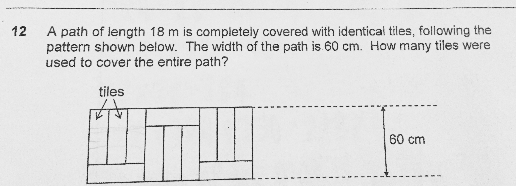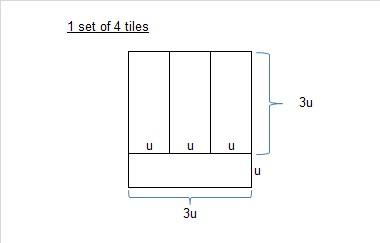# QuestionAnyone help me with attached question

Thanks3u + u = 60cm

4u = 60cm

u = 15cm

3u = 45cm

18m = 1800cm

1800 ÷ 45 = 40 sets of 4 tiles

40 × 4 = 160 tiles

0 Replies 1 Like ✔Accepted Answer

18 m = 1800 cm

area of entire path ——- 1800 x 60 = 108000

length of tiles ——- 3u

3u + 1u = 4u ——- 60

1u ——- 60/4 = 15

3u ——- 15 x 3 = 45

area of 1 tile ——- 45 x 15 = 675

108000/675 = 160

or

area of 4 tiles ——- 675 x 4 = 2700

108000/2700 = 40

40 x 4 = 160

Ans : 160 tiles.

0 Replies 1 Like